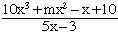Janna secondary question student Hi! I have two questions involving dividing polynomials by polynomials. Here's the first one: Two factors of 12a4 -39a2 + 8a - 8a3 + 12 are a - 2 and 2a + 1. Find the other factors. The other question I'm stuck on is: When 10x3 + mx2 - x + 10 is divided by 5x-3, the quotient is 2x2 + nx - 2 and the remainder is 4. Find the values of m and n. Thanks a lot for your help! Hi Janna, I would first calculate (a - 2)(2a + 1 ) = 2 a2 - a - 2. Since (a - 2) and (2a + 1) are factors of your quartic polynomial there are integers p, q and r so that 12a4 - 8a3 -39a2 + 8a + 12 = (2 a2 - a - 2)(p a2 + q + r) If you expand the right side by multiplication then the coefficient of a4 is 2p. But the coefficient of a4 on the left side is 12. Thus 2p = 12 and hence p = 6. In a similar fashion, the constant term on the right side is -2r and the constant on the left side is 12. Thus r = -6. Now find the coefficient of a on the right and left sides, set them equal and solve for q. Once you have p a2 + q + r you can factor it to answer the question asked. You can approach problem 2 is a similar way. Since the divisionyields 2x2 + nx - 2 with a remainder of 4, 10x3 + mx2 - x + 10 = (5x - 3)(2x2 + nx - 2) + 4 Expand the right side and equate coefficients. Cheers, Penny Go to Math Central